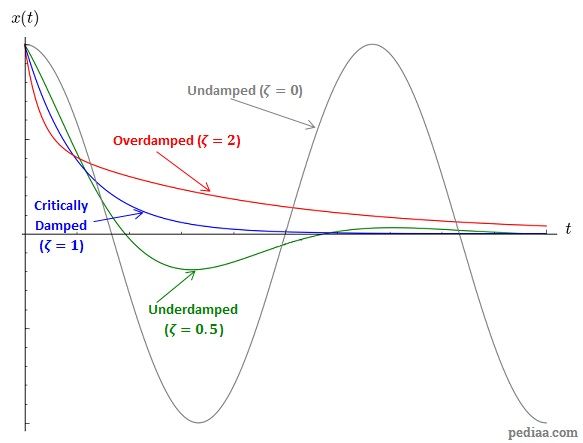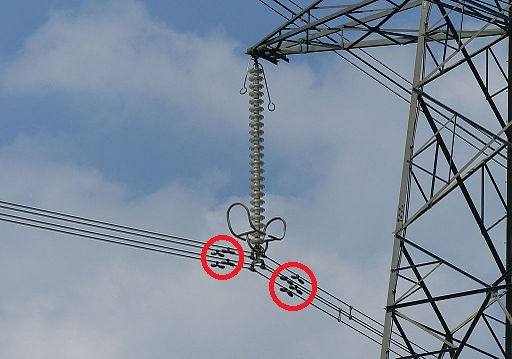# Difference Between Damped and Undamped Vibration

## Main Difference – Damped vs. Undamped Vibration

Damped and undamped vibration refer to two different types of vibrations. The main difference between damped and undamped vibration is that undamped vibration refer to vibrations where energy of the vibrating object does not get dissipated to surroundings over time, whereas damped vibration refers to vibrations where the vibrating object loses its energy to the surroundings.

## What is Undamped Vibration

In undamped vibrations, no resistive force acts on the vibrating object. As the object oscillates, the energy in the object is continuously transformed from kinetic energy to potential energy and back again, and the sum of kinetic and potential energy remains a constant value. In practice, it’s extremely difficult to find undamped vibrations. For instance, even an object vibrating in air would lose energy over time due to air resistance.

Let us consider an object undergoing simple harmonic motion. Here, the objet experiences a restoring force towards the equillibrium point, and the size of this force is proportional to displacement. If the displacement of the object is given by$x$, then for an object with mass$m$ in simple harmonic motion, we can write:$m\frac{\mathrm{d}^2x}{\mathrm{d}t^2}=-kx$

This is a differential equation. A solution to this equation can be written in the form:$x=A\:\mathrm{cos}\left( \omega t+\phi\right)$

Here,$\omega=\sqrt{\frac{k}{m}}$

If vibration is undamped, the object continues to oscillate sinusoidally.

## What is Damped Vibration

In damped vibrations, external resistive forces act on the vibrating object. The object loses energy due to resistance and as a result, the amplitude of vibrations decreases exponentially.

We can model the damping force to be directly proportional to the speed of the object at the time. If the constant of proportionality for the damping force is$b$, then we can write:$m\frac{\mathrm{d}^2x}{\mathrm{d}t^2}=-kx-b\frac{\mathrm{d}x}{\mathrm{d}t}$

The solution to this differential equation can be given in the form:$x=Ae^{\frac{-b}{2m}t}\:\mathrm{cos}\left( \omega' t+\phi\right)$.

Here, the$\omega'=\sqrt{\frac{k}{m}-\left( \frac{b}{2m}\right)^2}$.

We can write this as:$\omega'=\sqrt{1-\left( \frac{b}{2\sqrt{mk}}\right)^2}$.

Writing the equation in this form is useful because the quantity$\frac{b}{2\sqrt{mk}}$ can be used to determine the nature of a particular oscillation. Often, this quantity is called the damping coefficient,$\zeta$, i.e.$\zeta=\frac{b}{2\sqrt{mk}}$.

If$\zeta=1$, then we have critical damping. Under this condition, the oscillating object returns to its equilibrium position as soon as possible without completing any more oscillations. When$\zeta<1$, we have underdamping. In this case, the object continues to oscillate, but with an ever-reducing amplitude. For$\zeta>1$ the resistive forces are very strong. The object would not oscillate again, but the object is slowed down so much, that it goes towards the equilibrium much more slowly compared to an object that is critically damped. Overdamping is the name given to this type of scenario. When$\zeta=0$, there is no resistive force and the object is undamped. Theoretically, the object continues to carry out simple harmonic motion without any reduction in amplitude.

The graph below illustrates how the displacement of the object changes under these three different conditions:Damping under resistive forces with different damping constants

We can make use of damping in situations where we do not want something to vibrate. Cars consist of dampers that prevent the car from bobbing up and down repeatedly every time it falls into a pothole. Dampers are also found on bridges to prevent them from swaying due to wind. Tall buildings also sometimes have dampers to ensure that the building does not sway too much and topple during earthquakes. On power lines, “Stockbridge dampers” are used to ensure that the cables do not undergo large vibrations.Stockbridge dampers on a power line

## Difference Between Damped and Undamped Vibration

### Presence of Resistive Forces

In undamped vibrations, the object oscillates freely without any resistive force acting against its motion.

In damped vibrations, the object experiences resistive forces.

### Energy Loss

In undamped vibrations, the sum of kinetic and potential energies always gives the total energy of the oscillating object, and the value of its total energy does not change.

In damped vibrations, the total energy of the oscillating object decreases over time. This energy is dissipated as the object does work against the resistive forces.

### Value of the Damping Coefficient

For undamped vibrations,$\zeta=0$.

For damped vibrations,$\zeta>0$.

Image Courtesy:

“Stockbridge dampers on a 400 KV line near Castle Combe, England.” by Adrian Pingstone (Own work) [Public Domain], via Wikimedia Commons (Modified)